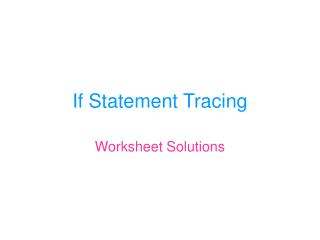Download PresentationIf Statement Tracing

# If Statement Tracing

Download Presentation## If Statement Tracing

- - - - - - - - - - - - - - - - - - - - - - - - - - - E N D - - - - - - - - - - - - - - - - - - - - - - - - - - -
##### Presentation Transcript

1. If Statement Tracing Worksheet Solutions

2. Question #1 If num = 10 Then score = score + 20 End If **no change to form

3. Question #2 If sum <> 0 Then score = score – 10 End If **no change to form

4. Question #3 If score >= 10 Then txtNumber.text = score Else txtNumber.text = num num = 0 End If

5. Question #4 If score < 100 Then txtNumber.text = score lblAnswer.caption = “you lose” Else txtNumber.text = num lblAnswer.caption = “you win” End If

6. Question #5 If price > 10 Then price = price * 1.15 txtNumber.text = price lblAnswer.caption = “15% tax” Else price = price * 0.5 txtNumber.text = price lblAnswer.caption = “50% off” End If

7. Question #6 If price < 10 Then sum = sum + score txtNumber.text = sum Else sum = sum + num txtNumber.text = sum End If

8. If price >= 5 Then num = num – 1 txtNumber.text = price ElseIf price >= 100 Then price = price + 1 txtNumber.text = price End If No change in variables or form Question #7

9. Question #8 If price < 2 Then lblAnswer.caption = “Cheap Price” ElseIf price > 3 Then lblAnswer.caption = “Adjusted Price” Else lblAnswer.caption = “Bad Price” End If

10. Question #9 price = price \ 10 If price > 2 Then price = price + 1 ElseIf price > 5 Then price = price – 1 Else sum = 0 End If lblAnswer.caption = sum

11. Question #10 If num = 0 Then lblAnswer.caption = “Zero” End If If score > 2 * num Then txtNumber.text = 2 * num Else txtNumber.text = num End If

12. Expressions – If Statements Written Assignment Solutions

13. Question #1 • 21 mod 5 = 1 • 32 \ 2 / 10 = 32 \ 0 = error • 32 / 2 \ 10 = 16 \ 10 = 1 • 100 / 2 \ 2 \ 2 = 50 \ 2 \ 2 = 25 \ 2 = 12 • 3 + 5 – 2 * 4 ^ 2 – 6 / 3 = 3 + 5 – 2 * 16 – 6 / 3 = 3 + 5 – 32 – 2 = -26

14. Question #2a If PRICE >= 50 then PRICE = PRICE – (PRICE * 0.3) End If **could also do Price = Price * 0.7

15. Question #2b If MARK < 50 Then COUNTER = COUNTER + 1 End If

16. Question #2c If WIN = true Then SCORE = SCORE +1 Else SCORE = SCORE -1 End If

17. Question # 2d If MARK >= 90 then MARK = MARK – (MARK * 0.1) End If **could also do MARK = MARK * 0.9

18. Question #2e If PRICE > 20 then PRICE = PRCIE + 2 ElseIf PRICE > 10 then PRICE = PRICE + 1 End If **be careful of the order of your statements!

19. Question # 2f If SCORE = 10 Then lblMessage.Caption = “Winner!” ElseIf SCORE < 0 Then lblMessage.Caption = “Loser!” End If

20. Question # 2g If DONATION > 2000 then CATEGORY = “Patron” ElseIf DONATION > 1000 then CATEGORY = “Helper” ElseIf DONATION >= 100 then CATEGORY = “Friend” Else CATEGORY = “MEMBER” End If **watch out for the order of your statements!

21. Question #2h If NUMBER mod 10 = 3 then NUMBER = NUMBER – 5 End If **when dividing any number by 10 (doesn’t matter the number of digits) you will get the last digit as the remainder!!

22. If fever > 98.7 Then health = “ill” ElseIf fever > 102.0 then health = “very ill” Else health = “normal” End If lblHealth.Caption = health b) Normal c) ill d) ill Question #3

23. Question #3 (continued) e) Any temperature over 98.7 degrees will give you ill as a comment. Any temperature below or equal to 98.7 degrees will give you normal as a comment. You will never get the comment very ill!!

24. Question #3 (continued) If fever > 102.0 then health = “very ill” ElseIf fever > 98.7 then health = “ill” Else health = “normal” End If lblHealth.Caption = health

25. Question #4 • Any number that is greater than 1 (2, 3, 4, ….) • Any number 14 or less (14, 13, 12, …) • This is true for absolutely any number • Any even number • Any number that has a tens digit of 0, or any single digit number

26. Question #5 1st method  If / ElseIf Commission = Price * 0.10 If Price > 30000 then Bonus = (Price – 20000) * 0.10 + 1000 ElseIf Price > 20000 then Bonus = (Price – 20000) * 0.10 End If Commission = Commission + Bonus

27. Question #5 2nd method  nested if Commission = Price * 0.10 If Price > 20000 then Bonus = (Price – 20000) * 0.10 If Price > 30000 then Bonus = Bonus + 1000 End If End If Commission = Commission + Bonus